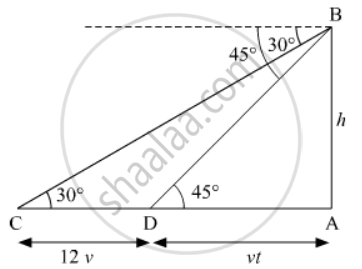# A Man Observes a Car from the Top of a Tower, Which is Moving Towards the Tower with a Uniform Speed. If the Angle of Depression of the Car Changes from 30° to 45° in 12 Minutes, Find the Time Taken by the Car Now to Reach the Tower. - Mathematics

A man observes a car from the top of a tower, which is moving towards the tower with a uniform speed. If the angle of depression of the car changes from 30° to 45° in 12 minutes, find the time taken by the car now to reach the tower.

#### Solution

Suppose AB be the tower of height h meters. Let C be the initial position of the car and let after 12 minutes the car be at D. It is given that the angles of depression at C and D are 30º and 45º respectively.

Let the speed of the car be v meter per minute. Then,

CD = distance travelled by the car in 12 minutes

CD = 12v metersSuppose the car takes t minutes to reach the tower AB from D. Then DA = vt meters

In DAB, we have

tan 45^@ = (AB)/(AD)

=> 1= h/(vt).....(i)

In ∆CAB, we have

tan 30^@ = (AB)/(AB)

=> 1/sqrt3 = h/(vt + 12v)

=> sqrt3t = vt + 12v .....(ii)

Substituting the value of h from equation (i) in equation (ii), we get

=> sqrt3t = t + 12

=> t = 12/(sqrt3 - 1) = 12/((sqrt3 - 1)) xx ((sqrt3 + 1))/((sqrt3 + 1)) = 6(sqrt3 + 1)

t = 16.39 minutes

t = 16 minutes 23 seconds

Concept: Heights and Distances
Is there an error in this question or solution?